U.S. Department of Transportation
1200 New Jersey Avenue, SE
Washington, DC 20590
202-366-4000

Federal Highway Administration Research and Technology
Coordinating, Developing, and Delivering Highway Transportation InnovationsThis report is an archived publication and may contain dated technical, contact, and link information
 Federal Highway Administration > Publications > Research Publications > 04097 > Measured Variability Of Southern Yellow Pine - Manual for LS-DYNA Wood Material Model 143
Publication Number: FHWA-HRT-04-097
Date: August 2007

# Measured Variability Of Southern Yellow Pine - Manual for LS-DYNA Wood Material Model 143

PDF files can be viewed with the Acrobat® Reader®

### 1.8 RATE EFFECTS

A number of approaches are available to accommodate strain-rate sensitivity. Simple approaches scale the input strengths or moduli as a function of strain rate. More sophisticated approaches use viscoplastic mechanisms. Viscoplastic mechanisms retard the development of plasticity, which allows the stress state to exceed the static failure surface. Viscoplastic mechanisms also increase the stiffness in conjunction with strength if plastic hardening is modeled in the prepeak regime.

Both types of formulations are easy to implement and are computationally efficient. Therefore, two methods are implemented. One method scales each strength as a function of strain rate, effectively expanding the failure surface. The second method is viscoplastic. Both methods are discussed here. However, the shifted surface method is the easiest to fit, and it is the only method that has been validated to date, so it is the only active option in the wood model at this time.

#### 1.8.1 High Strain-Rate Data

A common test for measuring strain-rate effects is the split Hopkinson pressure bar (SHPB). Hopkinson bar data for pine are shown in figure 19.(11) Here, the dynamic-to-static stress ratio is plotted as a function of impact velocity for both the parallel and perpendicular directions. Rate effects are more pronounced in the perpendicular direction than in the parallel direction.

Also plotted in figure 19 are theoretical curves derived from shock theory (i.e., conservation of mass and momentum across a shock front). The theoretical stress enhancement factors are: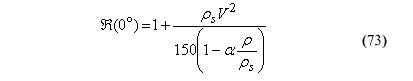where:

Â   =    ratio of dynamic-to-static stress

r    =    average wood density

rs  =    wood solid phase density

a   =    empirical shape factor for the stress-strain curve

Values of rs = 1500 kilograms per square meter (kg/m2) and a = 1.35 are reported by Reid and Peng(11) for wood and pine. For southern yellow pine with a density of r = 530 kg/m2 at 12-percent moisture content, the stress enhancement curves reduce to: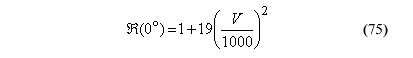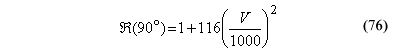where V is measured in m/s.

These curves indicate that the stress enhancement in the perpendicular direction is much greater than that in the parallel direction. However, the curves tend to underestimate the stress enhancement, particularly at low-impact velocities (see figure 19). To fit the rate-effect model parameters, the stress enhancement ratios need to be derived as a function of strain rate. To do this, impact velocity must be converted to strain rate. However, Reid and Peng(11) did not report this conversion.

Figure 19.

Hopkinson bar tests indicate that the measured strength of pine increases with impact velocity.

Source: Pergamon, Elsevier Science Ltd.(11)

Bragov and Lomunov also measured the dynamic properties of pine using the SHPB technique.(23) Stress-strain diagrams are depicted in figure 20. Data are measured parallel and perpendicular to the grain. In addition, two curve sets are shown for each direction, which correspond to two strain rates (approximately 1000 per second(s-1) and 500 s-1). The data indicate that both strength and parallel stiffness increase with strain rate.

Figure 20.

Hopkinson bar data indicate that strength and stiffness increase with strain rate.

Source: EDP Sciences.(23)

Parallel and perpendicular strength ratios versus strain rate are needed to fit the rate-effect model. Here, those ratios are estimated. Bragov and Lomunov report a static parallel compressive strength of XC = 41.4 MPa for pine.(23) This strength is used to calculate the parallel strength ratios from figure 20. Calculated values are Â(0°) = 2.3 at 1000 per second (s) and Â(0°) = 1.7 at 500/s. Bragov and Lomunov did not report a static perpendicular compressive strength for YC.(23) Therefore, one cannot directly estimate the perpendicular strength ratio from figure 20. Instead, the Reid and Peng velocity-strength ratio relationships in equation 76 are used to estimate the impact velocities and perpendicular strength ratios.(11) Impact velocities of 262 m/s and 192 m/s are derived from the parallel relationship in equation 76 for Â(0°) = 2.3 and Â(0°) = 1.7, respectively. Using these velocities, perpendicular strength ratios of Â(0°) = 9.0 and Â(0°) = 5.3 are derived from the perpendicular relationship in equation 76. These ratios are reported in table 11.

Table 11. Strength ratios versus strain rate derived from compressive rate-effect data.
Rate (s-1) Â(0°) Â(90°)
0
1.0
1.0
500
1.7
5.3
1000
2.3
9.0

#### 1.8.2 Shifted Surface Model Theory

Separate rate-effect formulations are modeled for the parallel and perpendicular modes because the Reid and Peng data indicate that dynamic strength enhancement is more pronounced in the perpendicular direction than in the parallel direction.(11) The rate-effect formulations increase strength with increasing strain rate by expanding each yield surface:

Parallel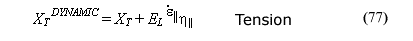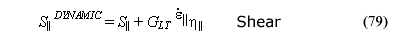Perpendicular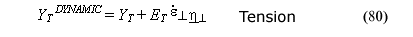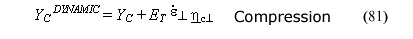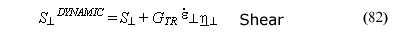where: X and Y =  static strengths XDYNAMIC and YDYNAMIC =  dynamic strengths C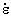h =  excess stress components

Excess stress components depend on the value of the fluidity parameter, h; the stiffness, C; and the effective strain rate,. When rate effects are requested via the flag IRATE = 1, dynamic strengths are used in place of the static strengths in the yield surface formulations (in equations 13 and 14, or in equations 44 and 45).

Four effective fluidity parameters are used (h||, hc||, h^, and hc^). Two parameters are needed for the parallel modes―one for the tensile and shear strengths, and a second for the compressive strength. Two parameters are also needed for the perpendicular modes.

Each effective fluidity parameter is formulated from two input parameters:

The two-parameter formulation allows the user to model a nonlinear variation in dynamic strength with strain rate.(24) Setting n|| = 0 or n^ = 0 allows the user to model a linear variation in dynamic strength with strain rate. The total number of input parameters is six. Three parameters are input for the parallel modes (h||, hc||, and n||) and three parameters are input for the perpendicular modes (h||, hc||, and n^).

Single-element simulations performed with and without rate effects (500/s) are shown in figure 21 for room-temperature clear wood at 12-percent moisture content. The dynamic-to-static strength ratio Â(0°) = 1.7, previously listed in table 11, was used to set the rate-effect parameters for the compressive simulation. These parameters are given in table 12. The plots in figure 21 indicate that different dynamic-to-static strength ratios naturally occur for the tension simulations than for the compressive simulations, even though h|| = hc||. This is because the tensile and compressive simulations have the same excess stress components, but different static strengths.

Figure 21.

These single-element simulations demonstrate the rate-effect behavior of the shifted surface formulation at 500/s.

Table 12. Default LS-DYNA rate-effect parameters that provide the dynamic-to-static compressive strength ratios listed in table 11 (based on units of milliseconds for time) for pine at 12-percent moisture content.
Wood Parallel Perpendicular
h|| hc|| n|| h^ hc^ n^
Pine
0.0045
0.0045
0.107
0.0962
0.0962
0.104
Fir
0.0045
0.0045
0.107
0.0962
0.0962
0.104

#### 1.8.3 Viscoplastic Model Theory

Viscoplastic algorithms allow the stress state to exceed the yield surface. The excess stress depends on the value of the fluidity parameter, h; the stiffness; and the strain rate. The viscoplastic (dynamic) stresses attained in uniaxial simulations are approximately those given in equations 77 through 82.

Single- and two-parameter viscoplastic formulations are available. The single-parameter formulation was developed by Duvaut and Lions and models a linear variation in strength ratio with strain rate.(25) This is accomplished by specifying a constant value for each fluidity parameter (independent of strain rate). However, a linear variation is not necessarily appropriate for wood, as demonstrated in figure 19. A two-parameter formulation was recently suggested by Murray to model a nonlinear variation in strength ratio with strain rate.(24) The two-parameter formulation was previously given in equations 83 through 86. The viscoplastic formulation uses the same input parameters as the shifted surface formulation.

The behavior of the viscoplastic model is shown in figure 22 for h = 0.0000094 s and four values of n. Here, the strength enhancement ratio (dynamic-to-static) has been plotted as a function of the strain rate. The smaller the value of n, the greater the strength enhancement at a given strain rate. The behavior of the two-parameter formulation reduces to that of the single-parameter formulation for n = 0. Thus, the Duvaut-Lions single-parameter model is a subset of the two-parameter model.

Figure 22.

Two-parameter viscoplastic model is flexible in fitting data.

The two-parameter viscoplastic update scheme is implemented as follows:

 where: Dt =  time step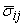and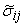=  viscid (dynamic) and inviscid (static) stress tensors, respectively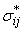=  trial elastic stress

At each time step,andare calculated and saved by the plasticity algorithm. Then, equations 87 through 91 are applied to update the viscous solution. The inviscid and instantaneous elastic solutions are obtained as limiting cases of the implementation. This algorithm is easy to implement because the plasticity algorithm is not modified. The viscoplastic formulation is separate from and follows the plasticity algorithm.

Separate formulations are implemented for the parallel (s11, s12, and s13) and perpendicular modes (s22, s33, and s23), because the Reid and Peng test data suggest that the rate effects depend on grain orientation.(11) The parallel stress components are updated with the parallel fluidity parameters, while the perpendicular stress components are updated with the perpendicular fluidity parameters. At this time, viscoplastic implementation requires only four parameters as input. These are h|| = hc|| and n|| for the parallel modes, and h^ = hc^ and n^ for the perpendicular modes. Therefore, one cannot specify separate dynamic overstresses for the tensile and compressive modes. Enhancement from four to six parameters could be conducted at a later date.

Single-element simulations performed with and without viscoplastic rate effects (500/s) are shown in figure 23 for room-temperature clear wood pine at 12-percent moisture content, using the input parameters specified in table 12.5 The primary difference between the viscoplastic simulations and the shifted surface simulations (figure 21) is that the viscoplastic tensile simulation is nonlinear to peak, whereas the shifted surface simulation is linear. This is because plastic strains are being calculated with the viscoplastic formulation, not the shifted surface formulation. The user has no control over the linearity or nonlinearity with either formulation.

Also note that the compressive simulations calculated with viscoplasticity attain the same dynamic peaks as those calculated with the shifted surface formulation. Different hardening parameters were used in each simulation, so the hardening is not identical. On the other hand, the tensile simulations calculated with the viscoplastic formulation attain lower peak strengths than those calculated with the shifted surface formulation. This is because damage accumulates in tension (not compression) and is applied to the stresses at the same time as viscoplasticity. Damage does not accumulate in tension during application of the shifted surface formulation.

5 Default hardening and softening parameters were not finalized at the time that these plots were made, so these figures cannot be reproduced using the current default material property values.

#### 1.8.4 Default Rate-Effect Parameters

Default rate-effect parameters for the shifted surface formulation were previously given in table 12. They provide the strength enhancement ratios listed in table 11. No rate-effect data are available for Douglas fir, so the model uses the same default parameters as southern yellow pine. The viscoplastic algorithm uses four of the six shifted surface parameters (the compressive fluidity parameters are ignored).

Figure 23.

These single-element simulations demonstrate the rate-effect behavior of the viscoplastic formulation at 500/s.

Federal Highway Administration | 1200 New Jersey Avenue, SE | Washington, DC 20590 | 202-366-4000
Turner-Fairbank Highway Research Center | 6300 Georgetown Pike | McLean, VA | 22101